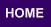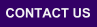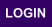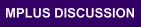Mplus
Monday
September 26, 2022Mplus Mplus at a GlanceGeneral DescriptionMplus Programs Pricing Version History System Requirements Platforms
 Analyses/ResearchMplus Examples Papers References
 Search

Chapter 9: Multilevel Modeling with Complex Survey Data

9.1: Two-level regression analysis for a continuous dependent variable with a random intercept (part a) ex9.1a ex9.1a.inp ex9.1a.dat mcex9.1a mcex9.1a.inp
9.1: Two-level regression analysis for a continuous dependent variable with a random intercept (part b) ex9.1b ex9.1b.inp ex9.1b.dat mcex9.1b mcex9.1b.inp
9.2: Two-level regression analysis for a continuous dependent variable with a random slope (part a) ex9.2a ex9.2a.inp ex9.2a.dat mcex9.2a mcex9.2a.inp
9.2: Two-level regression analysis for a continuous dependent variable with a random slope (part b) ex9.2b ex9.2b.inp ex9.2a.dat N/A N/A
9.2: Two-level regression analysis for a continuous dependent variable with a random slope (part c) ex9.2c ex9.2c.inp ex9.2c.dat mcex9.2c mcex9.2c.inp
9.3: Two-level path analysis with a continuous and a categorical dependent variable ex9.3 ex9.3.inp ex9.3.dat mcex9.3 mcex9.3.inp
9.4: Two-level path analysis with a continuous, a categorical, and a cluster-level observed dependent variable ex9.4 ex9.4.inp ex9.4.dat mcex9.4 mcex9.4.inp
9.5: Two-level path analysis with continuous dependent variables and random slopes ex9.5 ex9.5.inp ex9.5.dat mcex9.5 mcex9.5.inp
9.6: Two-level CFA with continuous factor indicators and covariates ex9.6 ex9.6.inp ex9.6.dat mcex9.6 mcex9.6.inp
9.7: Two-level CFA with categorical factor indicators and covariates ex9.7 ex9.7.inp ex9.7.dat mcex9.7 mcex9.7.inp
9.8: Two-level CFA with continuous factor indicators, covariates, and random slopes ex9.8 ex9.8.inp ex9.8.dat mcex9.8 mcex9.8.inp
9.9: Two-level SEM with categorical factor indicators on the within level and cluster-level continuous observed and random intercept factor indicators on the between level ex9.9 ex9.9.inp ex9.9.dat mcex9.9 mcex9.9.inp
9.10: Two-level SEM with continuous factor indicators and a random slope for a factor ex9.10 ex9.10.inp ex9.10.dat mcex9.10 mcex9.10.inp
9.11: Two-level multiple group CFA with continuous factor indicators ex9.11 ex9.11.inp ex9.11.dat mcex9.11 mcex9.11.inp
9.12: Two-level growth model for a continuous outcome (three-level analysis) ex9.12 ex9.12.inp ex9.12.dat mcex9.12 mcex9.12.inp
9.13: Two-level growth model for a categorical outcome (three-level analysis) ex9.13 ex9.13.inp ex9.13.dat mcex9.13 mcex9.13.inp
9.14: Two-level growth model for a continuous outcome (three-level analysis) with variation on both the within and between levels for a random slope of a time-varying covariate ex9.14 ex9.14.inp ex9.14.dat mcex9.14 mcex9.14.inp
9.15: Two-level multiple indicator growth model with categorical outcomes (three-level analysis) ex9.15 ex9.15.inp ex9.15.dat mcex9.15 mcex9.15.inp
9.16: Linear growth model for a continuous outcome with time-invariant and time-varying covariates carried out as a two-level growth model using the DATA WIDETOLONG command ex9.16 ex9.16.inp ex9.16.dat N/A N/A
9.17: Two-level growth model for a count outcome using a zero-inflated Poisson model (three-level analysis) ex9.17 ex9.17.inp ex9.17.dat mcex9.17 mcex9.17.inp
9.18: Two-level continuous-time survival analysis using Cox regression with a random intercept ex9.18 ex9.18.inp ex9.18.dat mcex9.18 mcex9.18.inp
9.19: Two-level mimic model with continuous factor indicators, random factor loadings, two covariates on within, and one covariate on between with equal loadings across levels (part 1) ex9.19part1 ex9.19part1.inp ex9.19.dat mcex9.19 mcex9.19.inp
9.19: Two-level mimic model with continuous factor indicators, random factor loadings, two covariates on within, and one covariate on between with equal loadings across levels (part 2) ex9.19part2 ex9.19part2.inp ex9.19.dat N/A N/A
9.19: Two-level mimic model with continuous factor indicators, random factor loadings, two covariates on within, and one covariate on between with equal loadings across levels (part 3) ex9.19part3 ex9.19part3.inp ex9.19.dat N/A N/A
9.20: Three-level regression for a continuous dependent variable ex9.20 ex9.20.inp ex9.20.dat mcex9.20 mcex9.20.inp
9.21: Three-level path analysis with a continuous and a categorical dependent variable ex9.21 ex9.21.inp ex9.21.dat mcex9.21 mcex9.21.inp
9.22: Three-level MIMIC model with continuous factor indicators, two covariates on within, one covariate on between level 2, one covariate on between level 3 with random slopes on both within and between level 2 ex9.22 ex9.22.inp ex9.22.dat mcex9.22 mcex9.22.inp
9.23: Three-level growth model with a continuous outcome and one covariate on each of the three levels ex9.23 ex9.23.inp ex9.23.dat mcex9.23 mcex9.23.inp
9.24:Regression for a continuous dependent variable using cross-classified data ex9.24 ex9.24.inp ex9.24.dat mcex9.24 mcex9.24.inp
9.25: Path analysis with continuous dependent variables using cross-classified data ex9.25 ex9.25.inp ex9.25.dat mcex9.25 mcex9.25.inp
9.26: IRT with random binary items using cross-classified data ex9.26 ex9.26.inp ex9.26.dat mcex9.26 mcex9.26.inp
9.27: Multiple indicator growth model with random intercepts and factor loadings using cross-classified data ex9.27 ex9.27.inp ex9.27.dat mcex9.27 mcex9.27.inp
9.28: Two-level regression analysis for a continuous dependent variable with a random intercept and a random residual variance ex9.28 ex9.28.inp ex9.28.dat mcex9.28 mcex9.28.inp
9.29: Two-level confirmatory factor analysis (CFA) with continuous factor indicators, covariates, and a factor with a random residual variance ex9.29 ex9.29.inp ex9.29.dat mcex9.29 mcex9.29.inp
9.30: Two-level time series analysis with a univariate first-order autoregressive AR(1) model for a continuous dependent variable with a random intercept, random AR(1) slope, and random residual variance ex9.30 ex9.30.inp ex9.30.dat mcex9.30 (step 1) mcex9.30.inp (step 1) mcex9.30 (step 2) mcex9.30.inp (step 2)
9.30: Two-level time series analysis with a univariate first-order autoregressive AR(1) model for a continuous dependent variable with a random intercept, random AR(1) and AR(2) slope, and random residual variance (part b) ex9.30b ex9.30b.inp ex9.30.dat none none
9.31: Two-level time series analysis with a univariate first-order autoregressive AR(1) model for a continuous dependent variable with a covariate, random intercept, random AR(1) slope, random slope, and random residual variance ex9.31 ex9.31.inp ex9.31.dat mcex9.31 mcex9.31.inp
9.32: Two-level time series analysis with a bivariate cross- lagged model for continuous dependent variables with random intercepts and random slopes ex9.32 ex9.32.inp ex9.32.dat mcex9.32 mcex9.32.inp
9.32: Two-level time series analysis with a bivariate cross- lagged model for continuous dependent variables with random intercepts and random slopes and random residual variances and covariance (part b) ex9.32b ex9.32b.inp ex9.32.dat none none
9.33: Two-level time series analysis with a first-order autoregressive AR(1) factor analysis model for a single continuous indicator and measurement error ex9.33 ex9.33.inp ex9.33.dat mcex9.33 mcex9.33.inp
9.33: Two-level, single-indicator measurement error model analyzed as a two-level ARIMA(1,0,1) = ARMA(1,1) with a random AR(1) (part b) ex9.33b ex9.33b.inp ex9.33.dat none none
9.34: Two-level time series analysis with a first-order autoregressive AR(1) confirmatory factor analysis (CFA) model for continuous factor indicators with random intercepts, a random AR(1) slope, and a random residual variance ex9.34 ex9.34.inp ex9.34.dat mcex9.34 mcex9.34.inp
9.35: Two-level time series analysis with a first-order autoregressive AR(1) IRT model for binary factor indicators with random thresholds, a random AR(1) slope, and a random residual variance ex9.35 ex9.35.inp ex9.35.dat mcex9.35 mcex9.35.inp
9.36: Two-level time series analysis with a bivariate cross-lagged model for two factors and continuous factor indicators with random intercepts and random slopes ex9.36 ex9.36.inp ex9.36.dat mcex9.36 mcex9.36.inp
9.37: Two-level time series analysis with a univariate first-order autoregressive AR(1) model for a continuous dependent variable with a covariate, linear trend, random slopes, and a random residual variance ex9.37 ex9.37.inp ex9.37.dat mcex9.37 mcex9.37.inp
9.38: Cross-classified time series analysis with a univariate first-order autoregressive AR(1) model for a continuous dependent variable with a covariate, random intercept, and random slope ex9.38 ex9.38.inp ex9.38.dat mcex9.38 mcex9.38.inp
9.38: Cross-classified time series analysis with a univariate first-order autoregressive AR(1) model for a continuous dependent variable with a covariate, random intercept, and random slope (part b) ex9.38b ex9.38b.inp ex9.38.dat none none
9.39: Cross-classified time series analysis with a univariate first-order autoregressive AR(1) model for a continuous dependent variable with a covariate, linear trend, and random slope ex9.39 ex9.39.inp ex9.39.dat mcex9.39 mcex9.39.inp
9.39: Cross-classified time series analysis with a univariate first-order autoregressive AR(1) model for a continuous dependent variable with a covariate, linear trend, and random slope (part b) ex9.39b ex9.39b.inp ex9.39.dat none none
9.40: Cross-classified time series analysis with a first-order autoregressive AR(1) confirmatory factor analysis (CFA) model for continuous factor indicators with random intercepts and a factor varying across both subjects and time ex9.40 ex9.40.inp ex9.40.dat mcex9.40 mcex9.40.inp
9.40: Cross-classified time series analysis with a first-order autoregressive AR(1) confirmatory factor analysis (CFA) model for continuous factor indicators with random intercepts, random factor loadings, and a factor varying across both subjects and time (part 2) ex9.40 (part 2) ex9.40.inp (part 2) ex9.40.dat (part 2) mcex9.27 mcex9.40.inp (part2)